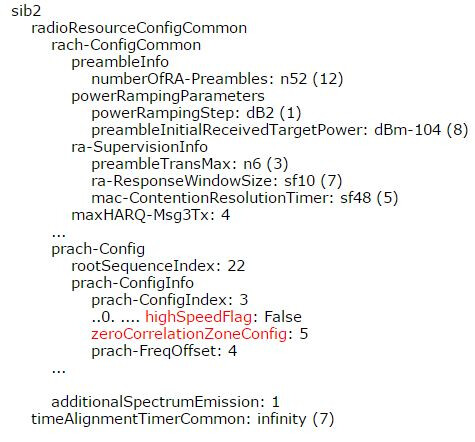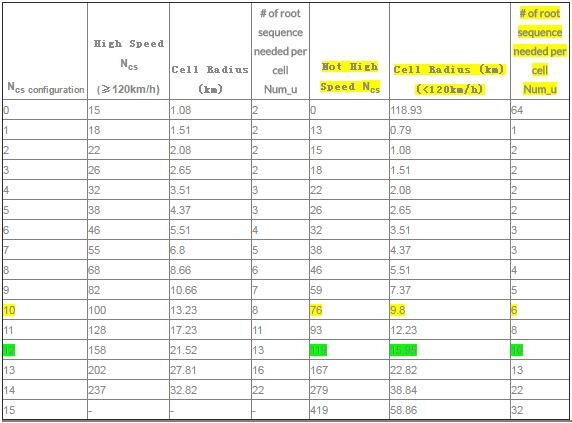# How to calculate the cell radius from PRACH format in 5g in Details

The calculation of the cell radius in 5G from the Physical Random Access CHannel (PRACH) format is similar to LTE technologies, but there are some differences due to the higher frequency bands used in 5G and the use of beamforming and Massive MIMO techniques. The following steps can be followed to calculate the cell radius from the PRACH format in 5G:

1. Determine the frequency band used by the cell. 5G uses frequency bands in the range of 600 MHz to 52.6 GHz, with higher frequency bands providing higher data rates but shorter coverage distances.
2. Calculate the wavelength of the signal using the formula: λ = c/f, where c is the speed of light and f is the frequency. In 5G, the wavelength is much shorter than in previous cellular technologies due to the use of higher frequency bands.
3. Find the duration of the PRACH preamble in seconds, which can be obtained from the 5G NR (New Radio) specifications. The PRACH preamble duration varies depending on the configuration, but it is typically in the range of a few microseconds.
4. Determine the propagation speed of the radio waves in the medium between the mobile device and the base station. This depends on the type of medium, such as air or a building material, and can be estimated or measured. In 5G, the propagation speed is affected by the higher frequency bands, which can cause more attenuation and reflection of the signals.
5. Calculate the cell radius using the formula: R = (c * T * √(n^2 - 1)) / (2 * n * f), where R is the cell radius, T is the duration of the PRACH preamble, n is the refractive index of the medium, and f is the frequency. In 5G, the calculation of the cell radius may also take into account the beamforming and Massive MIMO techniques used to increase the coverage and capacity of the network. Beamforming allows the base station to focus the signal towards the mobile device, which can increase the received signal power and reduce interference. Massive MIMO uses multiple antennas at the base station to improve the spatial efficiency of the communication, which can increase the capacity and coverage of the network.

Note that the refractive index of the medium can affect the propagation speed of the radio waves and should be taken into account in the calculation. It is also important to note that the cell radius in 5G may vary depending on the configuration of the network, the type of antenna used, and the interference level. The calculation provides an estimate of the cell radius, but the actual cell coverage may be affected by various factors such as terrain, obstacles, and mobility of the mobile devices.

Admin note: this post was updated with image below.

Credits:To calculate the cell radius from PRACH format in 5G, you can follow the steps below:

Determine the frequency band and subcarrier spacing (SCS) of the 5G network.
Identify the PRACH format being used in the network.
Obtain the PRACH configuration index (PCI) associated with the PRACH format.
Determine the root sequence index (RSI) associated with the PRACH format and PCI.
Use the following formula to calculate the cell radius:

Cell radius = c * (sqrt(P) / (2 * pi * SCS * sqrt(3))) * ((14 * Ncp) + 1) * (2^(RSI/2))

where:

• c = speed of light (3*10^8 m/s)
• P = maximum transmit power (in watts) of the UE
• SCS = subcarrier spacing (in Hz)
• Ncp = cyclic prefix length (either 0 or 1)
• RSI = root sequence index
• pi = 3.14159

Note: The value of P can be obtained from the UE’s power class, which is specified in the 5G standard.
Substitute the values of P, SCS, Ncp, and RSI into the formula and solve for the cell radius.
Keep in mind that this formula provides an estimate of the cell radius and may not be exact in all scenarios. Other factors such as interference and propagation conditions can also affect the coverage area of a cell.

This is not for 5G, but also helps in understanding.

Issue analysis of cell radius limited by PRACH parameters:

PRACH Parameter value is displayed in SIB2 during the Layer3 Analysis. During the drive test, find some place with good coverage for LTE system(RSRP=-106 dBm) but the UE cannot random access successfully because of defined value of prach parameter.

To enble this parameter make the following settings:Random Access Failure Reason Analysis

Below given snapshot shows that the UE RSRP is about -105 dBm at the distance of 10.2Km. UE is sending Random Access Request(MSG1) for many times but does not get any response from the eNB side. In this case we found that highspeedflag is False and zeroCorrelationZoneConfig is set to 10. It means cell radius is set to 9.8 km. When the UE will go beyond 9.8km, UE can send the Random Access Request but it will not get the response from the network side.

The cell access redius is determined by the PRACH Parameter(Ncs). Below mentioned table gives the relation between Ncs value and the cell radius.NCS Optimization

If ncs=12 (corresponding cell radius is 15.95 km) is more reasonable. So we suggest optimizing the ncs from 10 to 12.

Other PRACH parameters re-planning

According to table Table Ncs configuration, when ncs is changed, the number of root sequence needed per cell will change. That means the root sequence parameters need optimization too. The corresponding parameters are “Logical Root Sequence Start Number Used to Generate Prach Preamble” with short namerootSequenceIndex and “Transmission time configuration of random access preamble” with short name Prach Configuration Index. It is necessary to re-plan the rootSequenceIndex for each cell.

1 Like